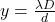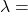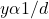## A double-slit diffraction pattern is formed on a distant screen. If the separation between the slits decreases, what happens to the distance

Question

A double-slit diffraction pattern is formed on a distant screen. If the separation between the slits decreases, what happens to the distance between interference fringes? Assume the angles involved remain small. A double-slit diffraction pattern is formed on a distant screen. If the separation between the slits decreases, what happens to the distance between interference fringes? Assume the angles involved remain small. The distance between interference fringes remains the same. The distance between interference fringes also decreases. The distance between interference fringes increases. The effect cannot be determined unless the distance between the slits and the screen is known.

in progress 0
2 months 2021-07-14T07:57:30+00:00 1 Answers 1 views 0

Option C. The distance between interference fringes increases.

Explanation:

The distance between interference fringes for small angles can be given by the formula:……….(1)

Where D = Distance between the slits and the screenthe wavelength of light

d = separation between the two slits

from the formula given in equation (1)It is obvious from the relationship above that the distance between interference fringes is inversely proportional to the separation between the slits.

Therefore, if the separation between slits is increased, the distance between interference fringes is increased.